# ARP协议简介

ARP 的中文名字是 “地址解析协议”，主要用在以太网中。 这里有一点需要明确的是， 所有的主机在互联网中通信的时候使用的是 IP 地址，而在以太网中通信时使用的却是硬件地 址（也就是常说的 MAC 地址）。

IP地址 硬件地址 类型
192.168.1.2 22:22:22:22:22:22 动态

1. 向目标发送一个ARP Request2. 如果目标主机处于活跃状态，它一定会回复一个ARP Reply3. 如果目标主机处于非活跃状态，它就不会给出任何回应# ARP实现的活跃主机扫描程序

这个程序有很多种方式可以实现，我们可以借助Scapy这个库来完成。

``ls(ARP())``

``ls(Ether())``

``````data =Ether(dst="ff:ff:ff:ff:ff:ff")/ARP(pdst="192.168.43.1")
srp(data,timeout=3)``````打开之后这就是我们看到的页面，然后我们运行我们在Pycharm中编写的代码：

``````data = Ether(dst="ff:ff:ff:ff:ff:ff") / ARP(pdst="192.168.43.1")
ans, unans = srp(data, timeout=3)
ans.summary()``````

``Ether / ARP who has 192.168.43.1 says 192.168.43.156 ==> Ether / ARP is at 72:b8:9a:72:f3:4a says 192.168.43.1``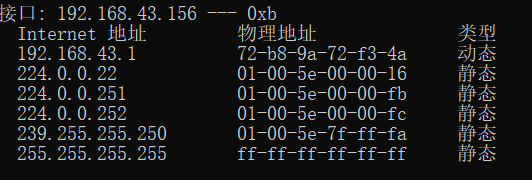按照之前的思路，需要对这个请求的回应进行监听，如果得到回应，那么证明目标在线，并打印输出这个机器的硬件地址。

``````data = Ether(dst="ff:ff:ff:ff:ff:ff") / ARP(pdst="192.168.43.1")
ans, unans = srp(data, timeout=3)
if ans:
ans.summary()
for s, r in ans:
print("目标MAC地址：" + str(r[Ether].src))``````

``````from scapy.all import *

ip = input("目标IP地址\范围：")
data = Ether(dst="ff:ff:ff:ff:ff:ff") / ARP(pdst=ip)
ans, unans = srp(data, timeout=3)
ans.summary()
if ans:
for s, r in ans:
ip = r[ARP].psrc
MAC = r[ARP].hwsrc
print("[*] 目标IP地址：%s" % ip)
print("[*] 目标MAC地址：%s" % MAC)
print("---------------")
else:
print("[!] 目标 %s 未在线！" % ip)``````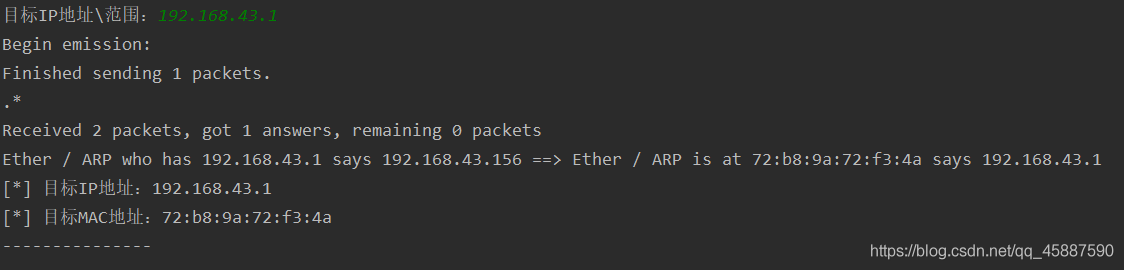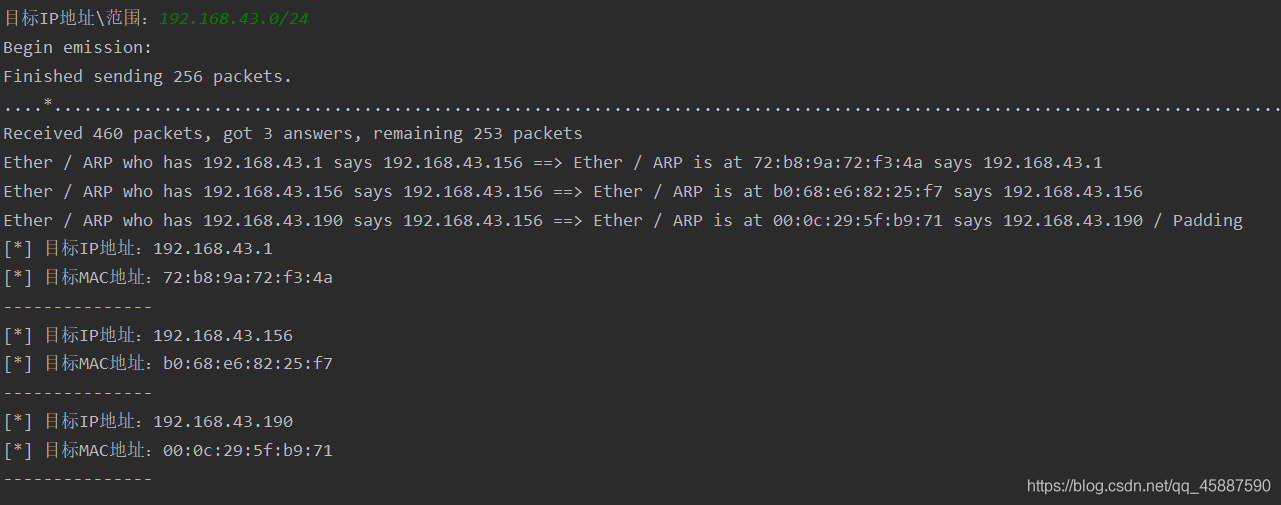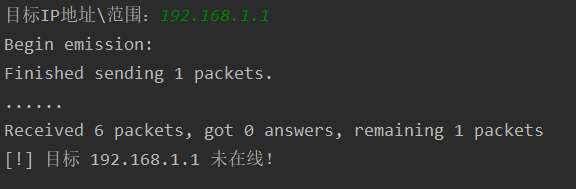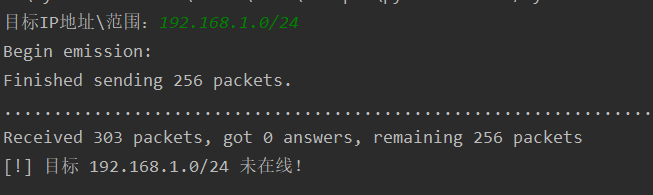在这里还是有一点缺陷的，要是能够把它列出来就好了。Kubrnete
Lv1

10

1

1